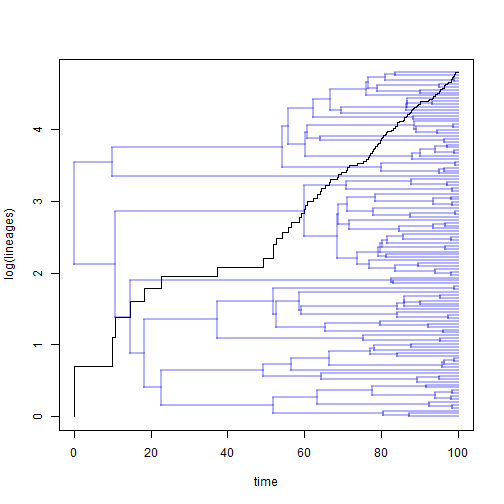## Thursday, November 9, 2017

### Comparing Yule & birth-death diversification models with an incomplete sampling fraction in phytools

In the following, I will demonstrate how to fit & compare a Yule (that is, pure-birth) and a birth-death diversification model in phytools.

First, here is our phylogeny:

``````library(phytools)
tree
``````
``````##
## Phylogenetic tree with 120 tips and 119 internal nodes.
##
## Tip labels:
##  t20, t21, t158, t147, t165, t196, ...
##
## Rooted; includes branch lengths.
``````
``````ltt(tree,show.tree=TRUE)
````````````## Object of class "ltt" containing:
##
## (1) A phylogenetic tree with 120 tips and 119 internal nodes.
##
## (2) Vectors containing the number of lineages (ltt) and branching times (times) on the tree.
##
## (3) A value for Pybus & Harvey's "gamma" statistic of 0.6307, p-value = 0.5282.
``````

Note that this is a simulated tree, but one in which I also simulated an incomplete sampling fraction of 0.6. That is to say, the true tree has 200 taxa, and the present tree contains what we assume to be a 60% random subset of these.

Fortunately, due to the work of Tanja Stadler & others (e.g., Stadler 2012) we can explicitly take this into account. Let's do so:

``````bd<-fit.bd(tree,rho=0.6)
bd
``````
``````##
## Fitted birth-death model:
##
## ML(b/lambda) = 0.085
## ML(d/mu) = 0.0443
## log(L) = -28.6273
##
## Assumed sampling fraction (rho) = 0.6
##
## R thinks it has converged.
``````
``````yule<-fit.yule(tree,rho=0.6)
yule
``````
``````##
## Fitted Yule model:
##
## ML(b/lambda) = 0.0578
## log(L) = -31.3279
##
## Assumed sampling fraction (rho) = 0.6
##
## R thinks it has converged.
``````

We can easily compare these alternative models using a LR-test (since they are nested) or AIC:

``````library(lmtest)
lrtest(yule,bd)
``````
``````## Likelihood ratio test
##
## Model 1: yule
## Model 2: bd
##   #Df  LogLik Df Chisq Pr(>Chisq)
## 1   1 -31.328
## 2   2 -28.627  1 5.401    0.02012 *
## ---
## Signif. codes:  0 '***' 0.001 '**' 0.01 '*' 0.05 '.' 0.1 ' ' 1
``````
``````AIC(yule,bd)
``````
``````##      df      AIC
## yule  1 64.65573
## bd    2 61.25469
``````

This shows that the birth-death model fits significantly better (or substantially better explains our data) than the Yule model.

Note that these methods duplicate the functionality of options that already existed in Rich Fitzjohn's popular diversitree R package.

E.g.:

``````library(diversitree)
lik.yule<-make.yule(tree,sampling.f=0.6)
yule<-find.mle(lik.yule,x.init=0.05)
yule
``````
``````## \$par
##     lambda
## 0.05785157
##
## \$lnLik
##  -31.32786
##
## \$counts
##  5
##
## \$code
##  1
##
##  -4.11319e-06
##
## \$method
##  "nlm"
##
## \$func.class
##  "yule"     "bd"       "dtlik"    "function"
##
## attr(,"func")
## Yule (pure birth) likelihood function:
##   * Parameter vector takes 1 elements:
##      - lambda
##   * Function takes arguments (with defaults)
##      - pars: Parameter vector
##      - condition.surv [TRUE]: Condition likelihood on survial?
##   * Phylogeny with 120 tips and 119 nodes
##      - Taxa: t20, t21, t158, t147, t165, t196, t146, t187, ...
##   * Reference:
##      - Nee et al. (1994) doi:10.1098/rstb.1994.0068
## R definition:
## function (pars, condition.surv = TRUE)
## attr(,"class")
##  "fit.mle.bd" "fit.mle"
``````
``````lik.bd<-make.bd(tree,sampling.f=0.6)
bd<-find.mle(lik.bd,x.init=c(0.07,0.02))
bd
``````
``````## \$par
##     lambda         mu
## 0.08494554 0.04425135
##
## \$lnLik
##  -28.62735
##
## \$counts
##  7
##
## \$code
##  1
##
##  -2.120260e-05  1.659828e-05
##
## \$method
##  "nlm"
##
## \$func.class
##  "bd"       "dtlik"    "function"
##
## attr(,"func")
## Constant rate birth-death likelihood function:
##   * Parameter vector takes 2 elements:
##      - lambda, mu
##   * Function takes arguments (with defaults)
##      - pars: Parameter vector
##      - condition.surv [TRUE]: Condition likelihood on survial?
##      - intermediates [FALSE]: Also return intermediate values?
##   * Phylogeny with 120 tips and 119 nodes
##      - Taxa: t20, t21, t158, t147, t165, t196, t146, t187, ...
##   * Reference:
##      - Nee et al. (1994) doi:10.1098/rstb.1994.0068
## R definition:
## function (pars, condition.surv = TRUE, intermediates = FALSE)
## attr(,"class")
##  "fit.mle.bd" "fit.mle"
``````
``````anova(yule,bd)
``````
``````##         Df   lnLik    AIC ChiSq Pr(>|Chi|)
## minimal  1 -31.328 64.656
## model 1  2 -28.627 61.255 5.401    0.02012 *
## ---
## Signif. codes:  0 '***' 0.001 '**' 0.01 '*' 0.05 '.' 0.1 ' ' 1
``````
``````AIC(yule,bd)
``````
``````##      df      AIC
## yule  1 64.65573
## bd    2 61.25469
``````

The results of these two methods should match.

Finally, it is probably worth noting the importance of getting sampling fraction right. For instance, the following shows what happens if I mistakenly think I have sampled 80% of the taxa in our group instead of 60%:

``````yule<-fit.yule(tree,rho=0.8)
yule
``````
``````##
## Fitted Yule model:
##
## ML(b/lambda) = 0.051
## log(L) = -29.589
##
## Assumed sampling fraction (rho) = 0.8
##
## R thinks it has converged.
``````
``````bd<-fit.bd(tree,rho=0.8)
bd
``````
``````##
## Fitted birth-death model:
##
## ML(b/lambda) = 0.0637
## ML(d/mu) = 0.023
## log(L) = -28.6273
##
## Assumed sampling fraction (rho) = 0.8
##
## R thinks it has converged.
``````
``````AIC(yule,bd)
``````
``````##      df      AIC
## yule  1 61.17793
## bd    2 61.25469
``````
``````lrtest(yule,bd)
``````
``````## Likelihood ratio test
##
## Model 1: yule
## Model 2: bd
##   #Df  LogLik Df  Chisq Pr(>Chisq)
## 1   1 -29.589
## 2   2 -28.627  1 1.9232     0.1655
``````

Now we estimate a much lower extinction rate in our birth-death model and there is no longer any evidence of significantly non-zero extinction!

The tree for this experiment was simulated using phytools as follows:

``````r<-(log(200)-log(2))/100
d<-0.03
b<-r+d
full.tree<-pbtree(n=200,b=b,d=d,t=100,extant.only=TRUE)
tree<-drop.tip(full.tree,sample(tree\$tip.label,80))
``````

That's it.